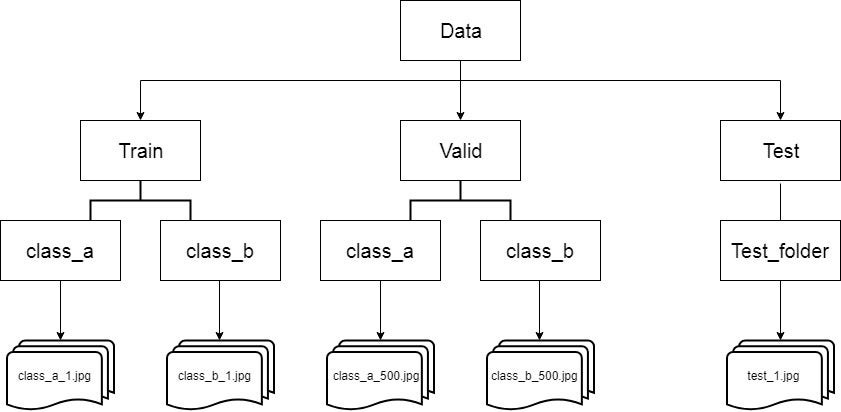# Tutorial on using Keras flow_from_directory and generatorsThe directory structure for a binary classification problem
`train_generator = train_datagen.flow_from_directory(    directory=r"./train/",    target_size=(224, 224),    color_mode="rgb",    batch_size=32,    class_mode="categorical",    shuffle=True,    seed=42)`
`valid_generator = valid_datagen.flow_from_directory(    directory=r"./valid/",    target_size=(224, 224),    color_mode="rgb",    batch_size=32,    class_mode="categorical",    shuffle=True,    seed=42)`
`test_generator = test_datagen.flow_from_directory(    directory=r"./test/",    target_size=(224, 224),    color_mode="rgb",    batch_size=1,    class_mode=None,    shuffle=False,    seed=42)`

# Let’s Train, evaluate and predict!

## Fitting/Training the model

`STEP_SIZE_TRAIN=train_generator.n//train_generator.batch_sizeSTEP_SIZE_VALID=valid_generator.n//valid_generator.batch_sizemodel.fit_generator(generator=train_generator,                    steps_per_epoch=STEP_SIZE_TRAIN,                    validation_data=valid_generator,                    validation_steps=STEP_SIZE_VALID,                    epochs=10)`

## Evaluate the model

`model.evaluate_generator(generator=valid_generator,steps=STEP_SIZE_VALID)`

## Predict the output

`STEP_SIZE_TEST=test_generator.n//test_generator.batch_sizetest_generator.reset()pred=model.predict_generator(test_generator,steps=STEP_SIZE_TEST,verbose=1)`
`predicted_class_indices=np.argmax(pred,axis=1)`
`labels = (train_generator.class_indices)labels = dict((v,k) for k,v in labels.items())predictions = [labels[k] for k in predicted_class_indices]`
`filenames=test_generator.filenamesresults=pd.DataFrame({"Filename":filenames,                      "Predictions":predictions})results.to_csv("results.csv",index=False)`

--

--